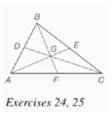Chapter 7.CR, Problem 24CR### Elementary Geometry for College St...

6th Edition
Daniel C. Alexander + 1 other
ISBN: 9781285195698

#### Solutions

Chapter
Section### Elementary Geometry for College St...

6th Edition
Daniel C. Alexander + 1 other
ISBN: 9781285195698
Textbook Problem
1 views

# Given: Δ A B C with medians A E , ¯ C D , ¯ B F ¯ Find: a) B G if B F = 18 b) G E if A G = 4 c) D G if C G = 4 3To determine

a)

To find: BG if BF=18.

Explanation

Given:

The triangle ABC with medians AE¯,CD¯,BF¯.

Theorem used:

The three medians of a triangle are concurrent at a point that is two-thirds the distance from any vertex to the midpoint of the opposite side.

Calculation:

We have BF=18

By the theorem, we get BF=32BG

To determine

b)

To find: GE if AG=4.

To determine

c)

To find: DG if CG=43.

### Still sussing out bartleby?

Check out a sample textbook solution.

See a sample solution

#### The Solution to Your Study Problems

Bartleby provides explanations to thousands of textbook problems written by our experts, many with advanced degrees!

Get Started

#### In Exercises 1-6, simplify the expression. 2. 2a23ab9b22ab2+3b3

Applied Calculus for the Managerial, Life, and Social Sciences: A Brief Approach

#### Find the derivatives of the functions in Problems 1-34. 19.

Mathematical Applications for the Management, Life, and Social Sciences

#### Find the limit. limx0sin5x3x

Single Variable Calculus: Early Transcendentals, Volume I

#### Convert the following percents to decimals. 76%

Contemporary Mathematics for Business & Consumers

#### Define applied research and basic research and identify examples of each.

Research Methods for the Behavioral Sciences (MindTap Course List)